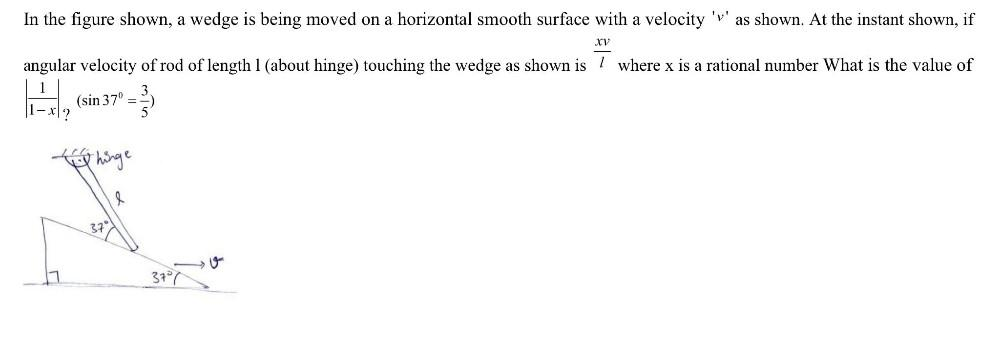Question:

# In the figure shown, a wedge is being moved on a horizontal smooth surface with a velocity 'v' as shown. At the instant shown, if angular velocity of rod of length xv/l where x is a rational number WhIn the figure shown, a wedge is being moved on a horizontal smooth surface with a velocity 'v' as shown. At the instant shown, if angular velocity of rod of length xv/l where x is a rational number What is the value of (1/1-x)? (sin37° = 3/5)